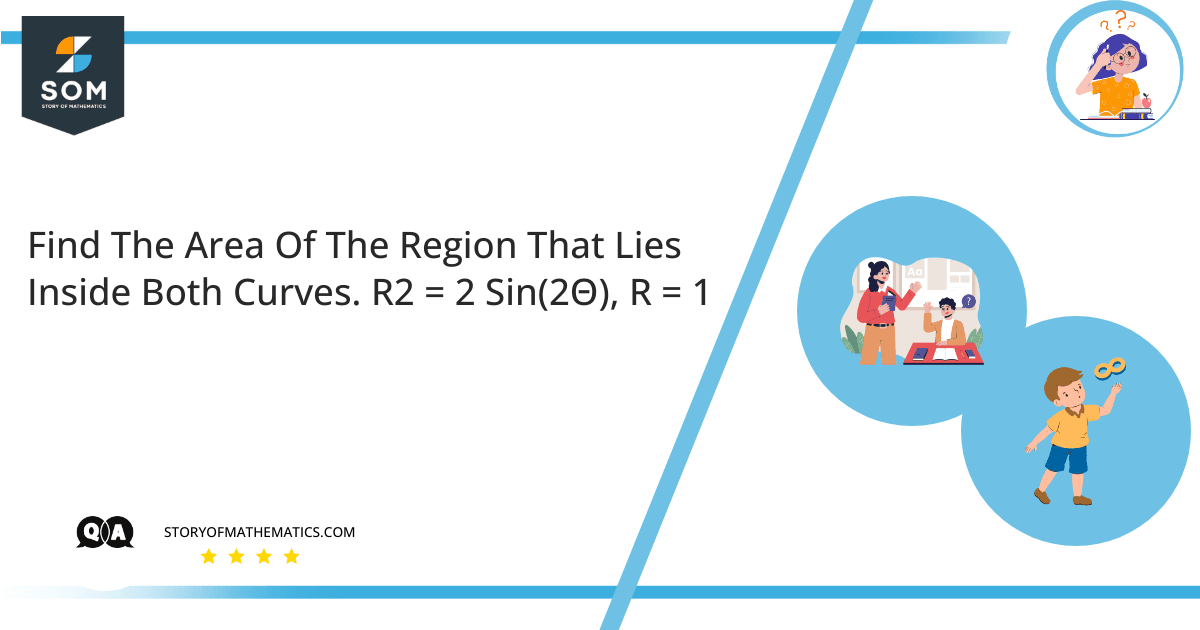# Find the area of the region that lies inside both curves.$r^{2}=50\sin(2\theta),\: r=5$

The article aims to find the region’s area under the given curves. Area under the curve is calculated by various methods, the most popular of which is the antiderivative method of finding the area.

Area under a curve can be found by knowing the curve’s equation, the boundaries of the curve, and the axis surrounding the curve. Generally, we have formulas to find areas of regular shapes like square, rectangle, quadrilateral, polygon, and circle, but there is no general formula to find the area under a curve. The process of integration helps solve the equation and find the required region.

Antiderivative methods are beneficial for finding regions of irregular planar surfaces. This article discusses how to find the area between two curves.

Area under the curve can be calculated in three simple steps.

First, we need to know the equation of the curve $(y = f(x))$, the limits over which the area is to be calculated, and the axis bounding the area.

Second, we need to find the integration (antiderivative) of the curve.

Finally, we need to apply an upper and lower bound to the integral response and take the difference to get the area under the curve.

$Area=\int_{a}^{b} y.dx$

$=\int_{a}^{b} f(x)dx$

$=[g(x)]_{a}^{b}$

$Area=g(b)-g(a)$

Area under the curve can be calculated using three ways. Also, which method is used to find the area under the curve depends on the need and available data inputs to find the area under the curve.

Step 1:

Consider the given curves $r^{2}=50\sin(2\theta),\: r=5$

The aim is to find the area of the region that lies under both curves.

From the curves:

$5^{2}=50\sin(2\theta)$

$25=50\sin(2\theta)$

$sin(2\theta)=\dfrac{1}{2}$

$2\theta=\dfrac{\pi}{6}, \dfrac{5\pi}{6}, \dfrac{13\pi}{6}, \dfrac{17\pi}{6}$

$\theta=\dfrac{\pi}{12}, \dfrac{5\pi}{12}, \dfrac{13\pi}{12}, \dfrac{17\pi}{12}$

Step 2:

The formula to find the area of the region under the curves is given by:

$A=\int_{a}^{b}\dfrac{1}{2}[f(\theta)]^2 \:d(\theta)$

The required area can be calculated by adding the area inside the cardioid between $\theta=0$ and $\theta=\dfrac{\pi}{4}$ from the area inside the circle $\theta=0$ to $\theta=\dfrac{\pi}{4}$.

Since the area is symmetric about $\theta=\dfrac{\pi}{4}$, the area can be calculated as:

$A=2[2\times \dfrac{1}{2}\int_{0}^{\dfrac{\pi}{12}}(\sqrt(50\sin(2\theta))^{2}d\theta +2\times \frac{1}{2} \int_{\dfrac{\pi}{12}}^{\dfrac{\pi}{4}} 5^{2} d\theta]$

$=2[\int-{0}^{\dfrac{\pi}{12}} 50\sin(2\theta)d\theta+\int_{\dfrac{\pi}{12}}^{\dfrac{\pi}{4}}25 \:d\theta]$

$=2[-\dfrac{50}{2}\cos(2\theta)|_{0}^{\dfrac{\pi}{12}}+25[|_{\dfrac{\pi}{12}}^{\dfrac{\pi}{4}}]$

$=2[-25(\cos\dfrac{\pi}{6}-\cos(0))+25(\dfrac{2\pi}{12}-\dfrac{\pi}{12})]$

$=2[-25(\dfrac{\sqrt 3}{2}-1)+25(\dfrac{2\pi}{12})]$

$=2(-\dfrac{25\sqrt 3}{2}+25+\dfrac{25\pi}{6})$

## Numerical Result

The area of the region under the curves $r^{2}=50\sin(2\theta),\: r=5$ is

$A=2(-\dfrac{25\sqrt 3}{2}+25+\dfrac{25\pi}{6})$

## Example

Calculate the area of the region that lies inside both curves.

$r^{2}=32\sin(2\theta),\: r=4$

Step 1:

Consider the given curves $r^{2}=32\sin(2\theta),\: r=4$

The aim is to find the area of the region that lies under both curves.

From the curves:

$4^{2}=32\sin(2\theta)$

$16=32\sin(2\theta)$

$sin(2\theta)=\dfrac{1}{2}$

$2\theta=\dfrac{\pi}{6}, \dfrac{5\pi}{6}, \dfrac{13\pi}{6}, \dfrac{17\pi}{6}$

$\theta=\dfrac{\pi}{12}, \dfrac{5\pi}{12}, \dfrac{13\pi}{12}, \dfrac{17\pi}{12}$

Step 2:

The formula to find the area of the region under the curves is given by:

$A=\int_{a}^{b}\dfrac{1}{2}[f(\theta)]^2 \:d(\theta)$

The required area can be calculated by adding the area inside the cardioid between $\theta=0$ and $\theta=\dfrac{\pi}{4}$ from the area inside the circle $\theta=0$ to $\theta=\dfrac{\pi}{4}$.

Since the area is symmetric about $\theta=\dfrac{\pi}{4}$, area can be calculated as:

$A=2[2\times \dfrac{1}{2}\int_{0}^{\dfrac{\pi}{12}}(\sqrt(32\sin(2\theta))^{2}d\theta +2\times \frac{1}{2} \int_{\dfrac{\pi}{12}}^{\dfrac{\pi}{4}} 4^{2} d\theta]$

$=2[\int-{0}^{\dfrac{\pi}{12}} 32\sin(2\theta)d\theta+\int_{\dfrac{\pi}{12}}^{\dfrac{\pi}{4}}16 \:d\theta]$

$=2[-\dfrac{32}{2}\cos(2\theta)|_{0}^{\dfrac{\pi}{12}}+16[|_{\dfrac{\pi}{12}}^{\dfrac{\pi}{4}}]$

$=2[-16(\cos\dfrac{\pi}{6}-\cos(0))+16(\dfrac{2\pi}{12}-\dfrac{\pi}{12})]$

$=2[-16(\dfrac{\sqrt 3}{2}-1)+16(\dfrac{2\pi}{12})]$

$=2(-\dfrac{16\sqrt 3}{2}+16+\dfrac{16\pi}{6})$

The area of the region under the curves $r^{2}=32\sin(2\theta),\: r=4$ is

$A=2(-\dfrac{16\sqrt 3}{2}+16+\dfrac{16\pi}{6})$